# Determine if a workbook is already open using VBA in Microsoft Excel

In Excel we can determine whether workbook is close or open through VBA code. Below is the VBA code and process which you need to paste in the code module of the file.

1. Open Excel
2. Press ALT + F11
3. VBA Editor will OPEN
4. Click anywhere in the Project Window
5. Click on Insert
6. Click on Module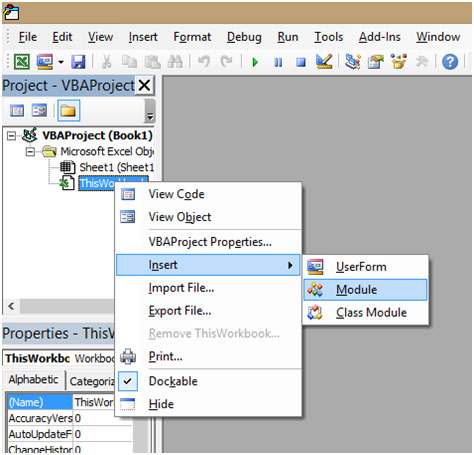7. In the Code Window, Copy and Paste the below mentioned Code

Function WorkbookOpen(WorkBookName As String) As Boolean
' returns TRUE if the workbook is open
WorkbookOpen = False
On Error GoToWorkBookNotOpen
If Len(Application.Workbooks(WorkBookName).Name) > 0 Then
WorkbookOpen = True
Exit Function
End If
WorkBookNotOpen:
End Function

8. Once this is pasted, go to the Excel file
9. Select cell A1
10. Type =work
11. You can see that =workbookopen is visible like a formula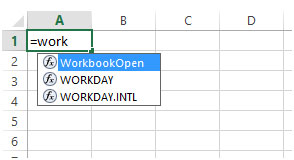12. Initially it was not there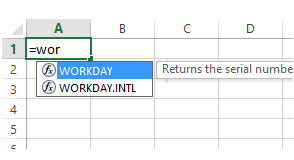13. Write the formula =WorkbookOpen("test1.xlsx")
14. Here the workbook "test1.xlsx" is used as an example. This file is not open.
15. As this file is not open it will show as “FALSE”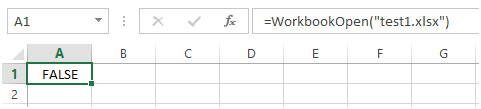16. Select cell A3
18. Here the workbook "determine-if-a-workbook-is-already-open-using-vba-in-microsoft-excel.xlsx" is used as an example. This file is open.
19. As this file is open, it will show as “TRUE”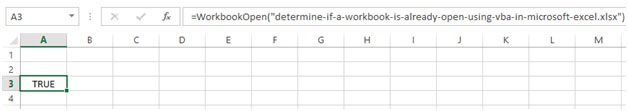This is how we can determine if the workbook is already open or not using VBA in Microsoft Excel 2010 and Microsoft Excel 2013.